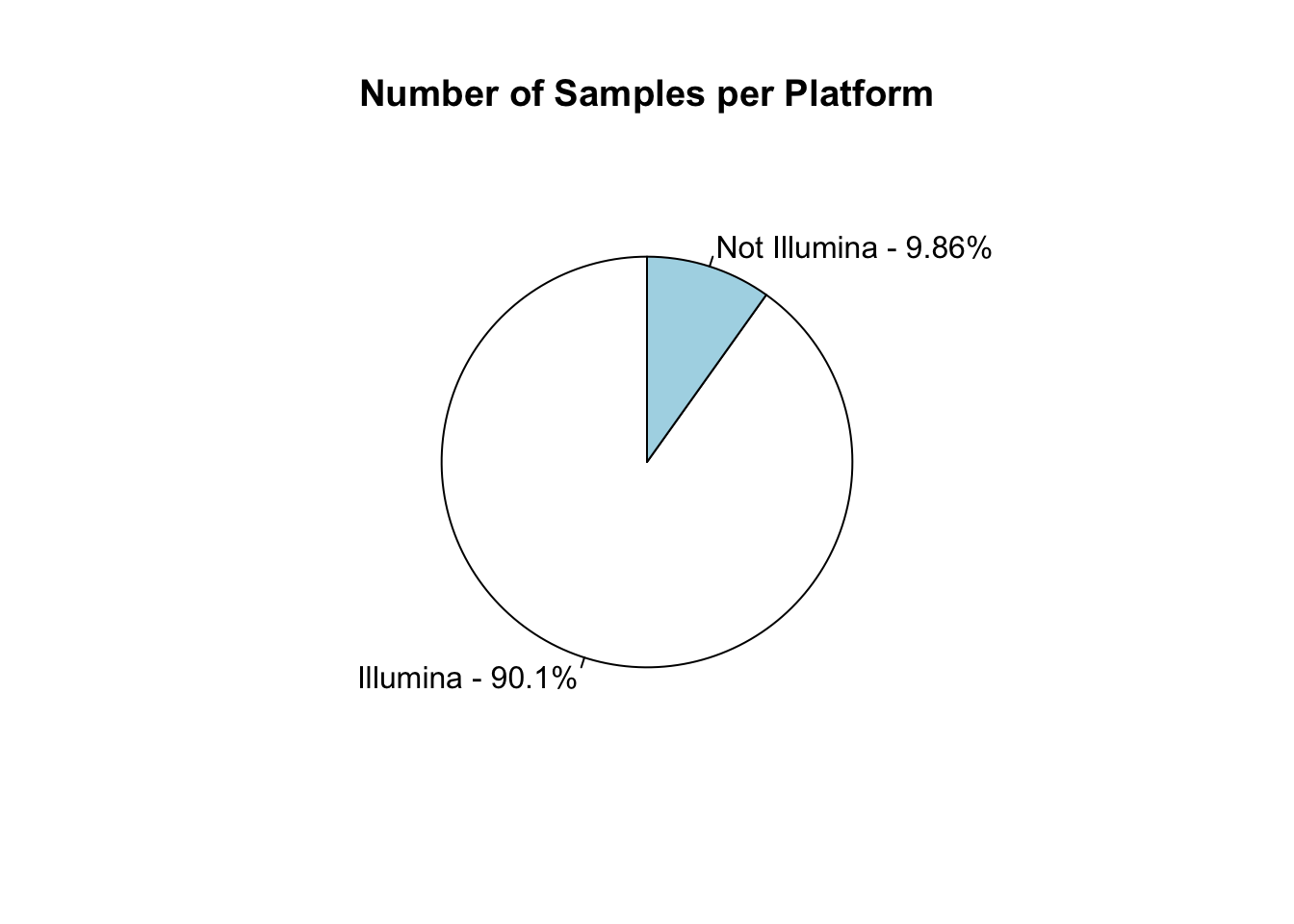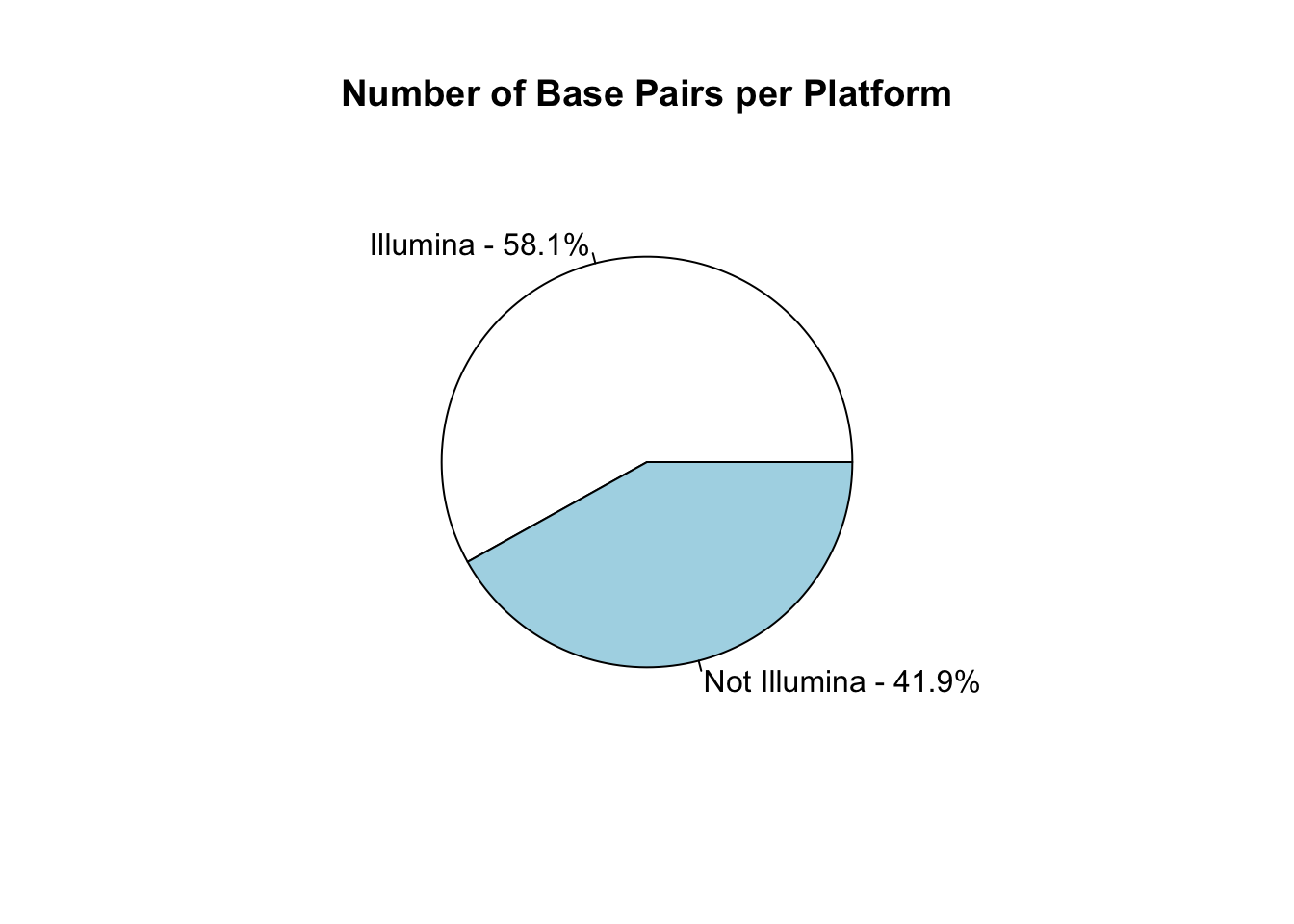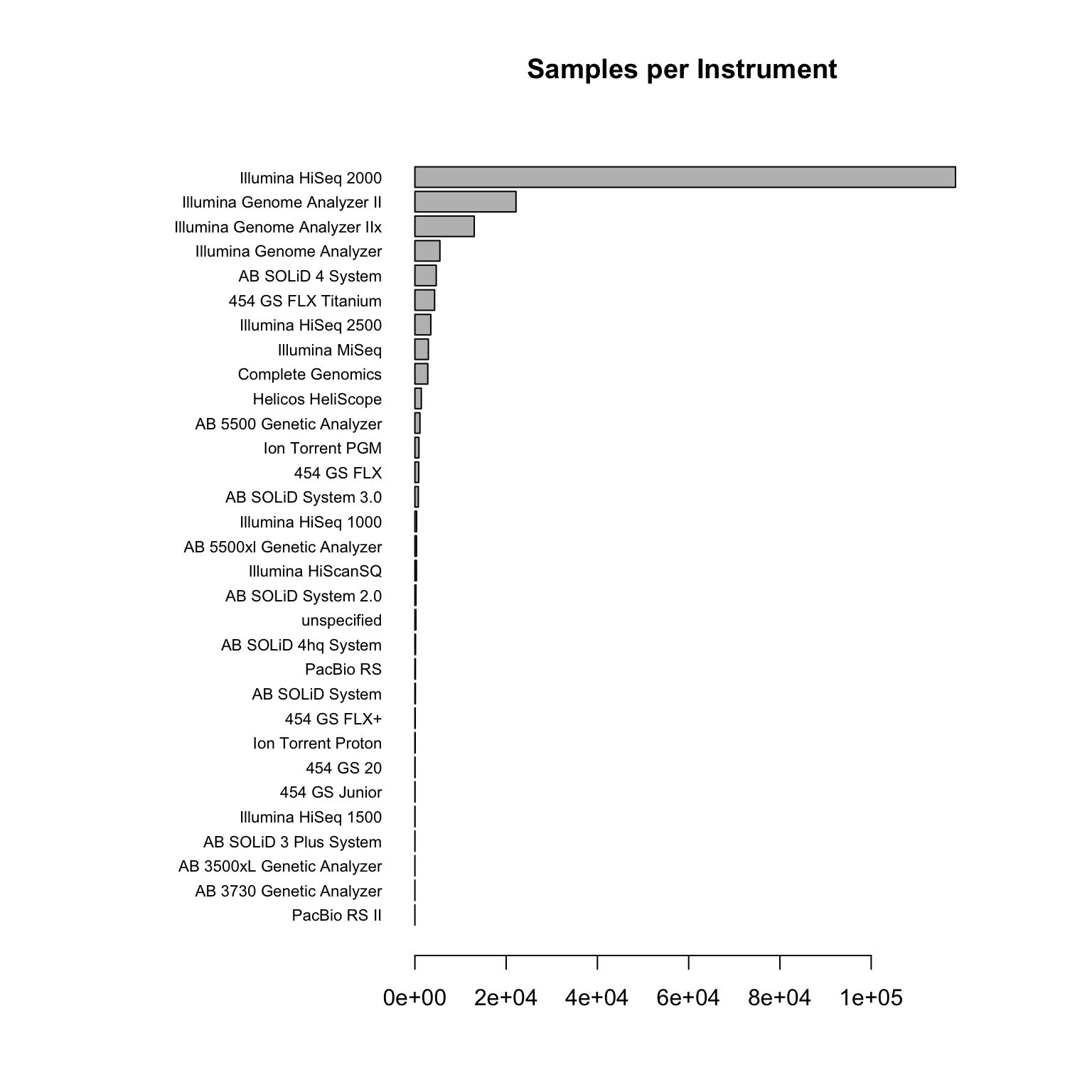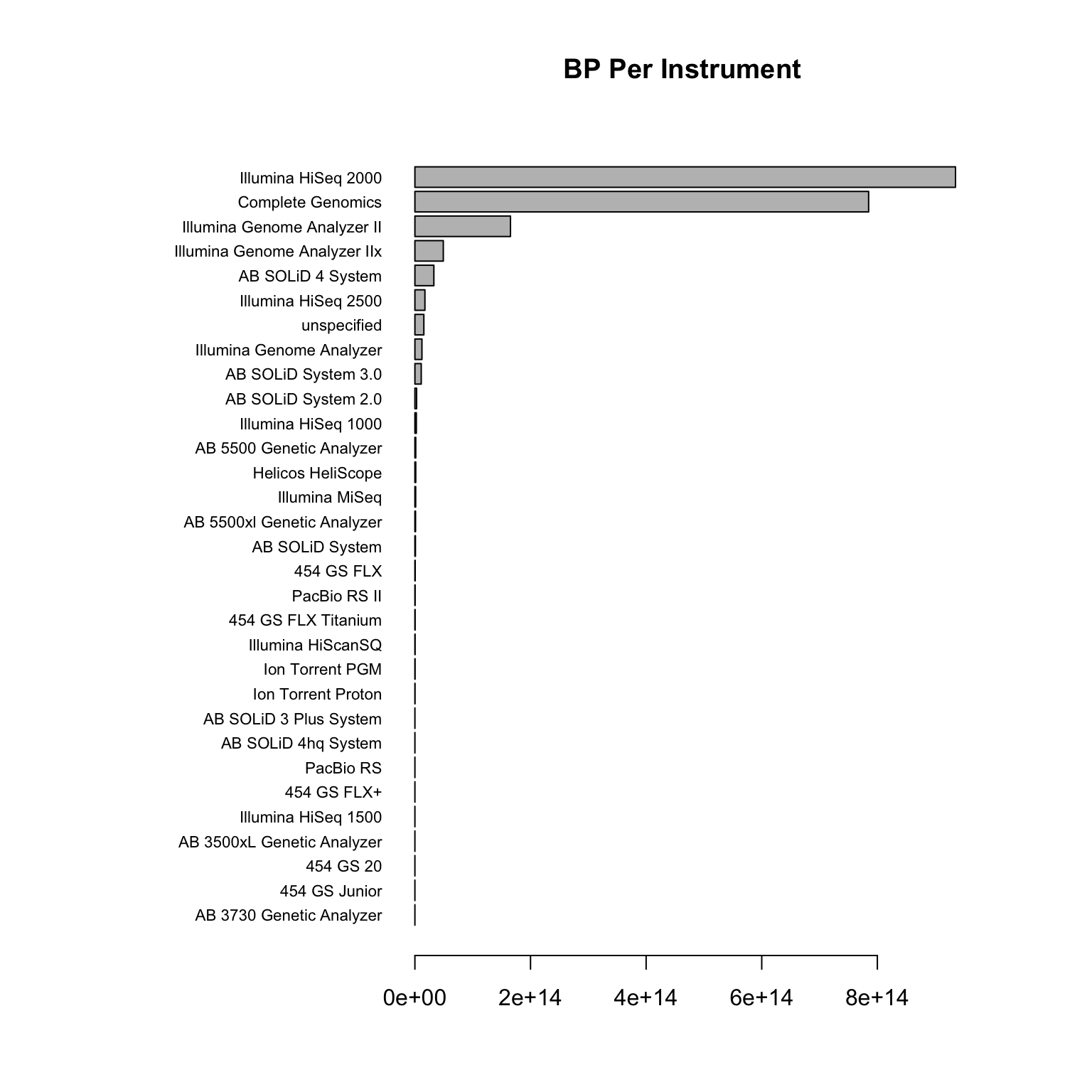The Sequence Read Archive (SRA) contains the raw DNA-sequencing data for many published datasets. We were interested in which sequencing platforms were prevalent, or rather, how prevalent Illumina Sequencing is. We were specifically interested in Human data, notably Whole Genome Sequencing (WGS).

``````# SETUP
library('scales')``````

To get at the metadata contained within the SRA, I did a search for all human samples - `"homo sapiens"[Organism]`. This returns 184586 results at the time of writing. The summaries of these search results can be downloaded as a `.csv` file, which is used in this analysis.

``````# Load in the data

# Look at the column headers
colnames(data)``````
``````##   "Experiment.Accession"   "Experiment.Title"
##   "Organism.Name"          "Instrument"
##   "Submitter"              "Study.Accession"
##   "Study.Title"            "Sample.Accession"
##   "Sample.Title"           "Total.Size..Mb"
##  "Total.RUNs"             "Total.Spots"
##  "Total.Bases"            "FTP.Path.to.Experiment"
##  "Library.Name"           "Library.Strategy"
##  "Library.Source"         "Library.Selection"``````
``````# Split up by Illumina and Not Illumina
illumina_machines <- c('Illumina Genome Analyzer', 'Illumina Genome Analyzer II', 'Illumina Genome Analyzer IIx',
'Illumina HiScanSQ', 'Illumina HiSeq 1000', 'Illumina HiSeq 1500', 'Illumina HiSeq 2000',
'Illumina HiSeq 2500', 'Illumina MiSeq')
total_illumina <- data[(data\$Instrument %in% illumina_machines),]
total_not_illumina <- data[!(data\$Instrument %in% illumina_machines),]``````

# Initial Overview

We’re most interested in the number of samples and the total number of base pairs sequenced - these give a feel of the dominancy of certain platforms.

``````# Count samples and base pairs
num_samples <- nrow(data)
num_samples.illumina <- nrow(total_illumina)
num_samples.not_illumina <- nrow(total_not_illumina)
num_bp <-sum(data\$Total.Bases, na.rm=TRUE)
num_bp.illumina <- sum(total_illumina\$Total.Bases, na.rm=TRUE)
num_bp.not_illumina <- sum(total_not_illumina\$Total.Bases, na.rm=TRUE)

# What numbers are we talking about here?
num_samples``````
``##  184496``
``num_bp``
``##  2.037e+15``
``````# Plot the number of samples for Illumina / Not Illumina
par(mai=c(1,1,1,1))
pie(c(num_samples.illumina, num_samples.not_illumina), init.angle=90,
labels=c(paste('Illumina -', percent(num_samples.illumina/num_samples)),
paste('Not Illumina -', percent(num_samples.not_illumina/num_samples))))
title("Number of Samples per Platform")````````````# Plot the total base pairs sequenced for Illumina / Not Illumina
pie(c(num_bp.illumina, num_bp.not_illumina), init.angle=0,
labels=c(paste('Illumina -', percent(num_bp.illumina/num_bp)),
paste('Not Illumina -', percent(num_bp.not_illumina/num_bp))))
title("Number of Base Pairs per Platform")``````## Hang on, what was that?

The above plot is really surprising! The vast majority of samples are sequences using Illumina machines, but when looking at the total number of base pairs this drops significantly.

# Explaining the skew in percentage of base pairs

``````# Back to the original data - plot number of samples for all instruments
par(mai=c(1,3,1,1))
samples_by_instrument <- table(data\$Instrument)
samples_by_instrument <- sort(samples_by_instrument)
barplot(samples_by_instrument, horiz=TRUE, las=1, main="Samples per Instrument", cex.names=0.7)````````````# Plot the total base pairs for all instruments
bp_by_instrument <- rowsum(data\$Total.Bases, data\$Instrument, na.rm = TRUE)
bp_by_instrument <- bp_by_instrument[order(bp_by_instrument[,1]),] # sort by frequency
barplot(bp_by_instrument, horiz=TRUE, las=1, main="BP Per Instrument", cex.names=0.7)``````Ok, So it looks like this discrepancy is caused by a handful of samples sequenced using the `Complete Genomics` platform. What kinds of studies were these?

``````# Split this up for library types
# I should group the low frequency types into "other". For now, it's just
# sorted by frequency so ignore the stuff at the bottom of the legend.
par(mai=c(1,3,1,1))
samples_type_per_instrument <- table(data\$Library.Strategy, data\$Instrument)
samples_type_per_instrument <- samples_type_per_instrument[order(rowSums(samples_type_per_instrument),decreasing=TRUE),order(colSums(samples_type_per_instrument))]
library('RColorBrewer')
colours<-brewer.pal(11, 'Spectral')
cols<-colorRampPalette(colours)(nrow(samples_type_per_instrument))
barplot(samples_type_per_instrument, horiz=TRUE, las=1, main="Samples per Instrument", col=colours, cex.names=0.7)
legend("bottomright", rownames(samples_type_per_instrument), fill=colours, cex=0.7)``````